Cubes And Dice Quiz I, Non Verbal Reasoning

# Cubes And Dice Quiz I, Non Verbal Reasoning - SSC CGL

Test Description

## 25 Questions MCQ Test Non Verbal Reasoning - Cubes And Dice Quiz I, Non Verbal Reasoning

Cubes And Dice Quiz I, Non Verbal Reasoning for SSC CGL 2023 is part of Non Verbal Reasoning preparation. The Cubes And Dice Quiz I, Non Verbal Reasoning questions and answers have been prepared according to the SSC CGL exam syllabus.The Cubes And Dice Quiz I, Non Verbal Reasoning MCQs are made for SSC CGL 2023 Exam. Find important definitions, questions, notes, meanings, examples, exercises, MCQs and online tests for Cubes And Dice Quiz I, Non Verbal Reasoning below.
Solutions of Cubes And Dice Quiz I, Non Verbal Reasoning questions in English are available as part of our Non Verbal Reasoning for SSC CGL & Cubes And Dice Quiz I, Non Verbal Reasoning solutions in Hindi for Non Verbal Reasoning course. Download more important topics, notes, lectures and mock test series for SSC CGL Exam by signing up for free. Attempt Cubes And Dice Quiz I, Non Verbal Reasoning | 25 questions in 50 minutes | Mock test for SSC CGL preparation | Free important questions MCQ to study Non Verbal Reasoning for SSC CGL Exam | Download free PDF with solutions
 1 Crore+ students have signed up on EduRev. Have you?
Cubes And Dice Quiz I, Non Verbal Reasoning - Question 1

### Directions to Solve The sheet of paper shown in the figure (X) given on the left hand side, in each problem, is folded to form a box. Choose from amongst the alternatives (1), (2), (3) and (4), the boxes that are similar to the box that will be formed. Question - Choose the box that is similar to the box formed from the given sheet of paper (X).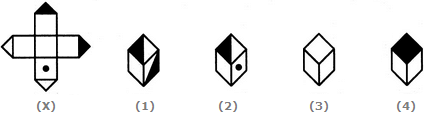Detailed Solution for Cubes And Dice Quiz I, Non Verbal Reasoning - Question 1
The fig. (X) is similar to the Form VI. So, when a cube is formed by folding the sheet shown in fig. (X), then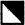is one of the faces of the cube. However, the cube in fig. (1) has two such faces and fig. (4) has a face which is completely shaded. So, these two cubes cannot be formed. Hence, only the cubes in figures (2) and (3) can be formed.
Cubes And Dice Quiz I, Non Verbal Reasoning - Question 2

### Directions to Solve The sheet of paper shown in the figure (X) given on the left hand side, in each problem, is folded to form a box. Choose from amongst the alternatives (1), (2), (3) and (4), the boxes that are similar to the box that will be formed. Question - Choose the box that is similar to the box formed from the given sheet of paper (X).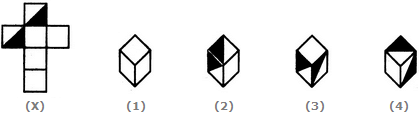Detailed Solution for Cubes And Dice Quiz I, Non Verbal Reasoning - Question 2
The fig. (X) is similar to the Form I. So, when the sheet shown in fig. (X) is folded to form a cube then one of the two half-shaded faces lies opposite to one of the blank faces and the other half-shaded face lies opposite to another blank face. The two remaining blank faces lie opposite to each other. Thus, both the cubes shown in figures (1).and (4) can be formed when the sheet shown in fig. (X) is folded. Also, though the cubes shown in figures (2) and (3) have faces that can appear adjacent to each other but the cube formed by folding the sheet in fig. (X) cannot be rotated to form either of the two. Hence, the cubes in figures (2) and (3) cannot be formed.
Cubes And Dice Quiz I, Non Verbal Reasoning - Question 3

### Directions to Solve The sheet of paper shown in the figure (X) given on the left hand side, in each problem, is folded to form a box. Choose from amongst the alternatives (1), (2), (3) and (4), the boxes that are similar to the box that will be formed. Question - How many dots lie opposite to the face having three dots, when the given figure is folded to form a cube?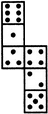Detailed Solution for Cubes And Dice Quiz I, Non Verbal Reasoning - Question 3
The given figure is similar to Form V. Therefore, when this figure is folded to form a cube then the face bearing six dots will lie opposite the face bearing three dots.
Cubes And Dice Quiz I, Non Verbal Reasoning - Question 4

The sheet of paper shown in the figure (X) given on the left hand side, in each problem, is folded to form a box. Choose from amongst the alternatives (1), (2), (3) and (4), the boxes that are similar to the box that will be formed.

Question -

Choose the box that is similar to the box formed from the given sheet of paper (X).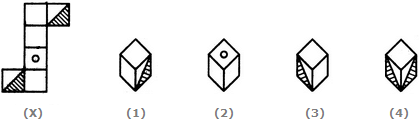Detailed Solution for Cubes And Dice Quiz I, Non Verbal Reasoning - Question 4
The fig. (X) is similar to Form II. So, when the sheet shown in fig. (X) is folded to form a cube then the two half-shaded faces lie opposite to each other, the face bearing a circle lies opposite to one of the two blank faces and the two remaining blank faces lie opposite to each other. Therefore, the cubes shown in fig. (4) which has the two half-shaded faces adjacent to each other, cannot be formed by folding the sheet shown in fig. (X). Also, the cube shown in fig. (2) has the face bearing a circle adjacent to two blank faces. This is not possible since there is one blank face opposite to the circle and one blank face opposite to the third blank face. Hence, only the cubes in figures (1) and (3) can be formed.
Cubes And Dice Quiz I, Non Verbal Reasoning - Question 5

The sheet of paper shown in the figure (X) given on the left hand side, in each problem, is folded to form a box. Choose from amongst the alternatives (1), (2), (3) and (4), the boxes that are similar to the box that will be formed.

Question -

Choose the box that is similar to the box formed from the given sheet of paper (X).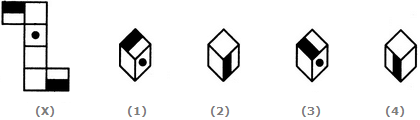Detailed Solution for Cubes And Dice Quiz I, Non Verbal Reasoning - Question 5
The fig. (X) is similar to Form II. So, when a cube is formed by folding the sheet shown in fig. (X), then the two half-shaded faces lie opposite to each other and one. of the three blank faces appears opposite to the face bearing a dot. Clearly, each one of the four cubes shown in figures (1), (2), (3) and (4) can be formed by folding the sheet shown in fig. (X).
Cubes And Dice Quiz I, Non Verbal Reasoning - Question 6

The sheet of paper shown in the figure (X) given on the left hand side, in each problem, is folded to form a box. Choose from amongst the alternatives (1), (2), (3) and (4), the boxes that are similar to the box that will be formed.

Question -

Choose the box that is similar to the box formed from the given sheet of paper (X).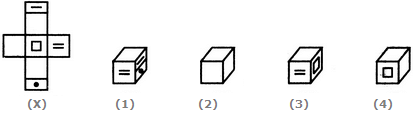Detailed Solution for Cubes And Dice Quiz I, Non Verbal Reasoning - Question 6
When the sheet in fig. (X) is folded, then one of the faces of the cube formed will be of the form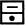and this face will lie opposite the face bearing a square. Also, one of the blank faces lies opposite another blank face and the third blank face lies opposite the face bearing an '=' sign. Clearly, all the three blank faces cannot appear adjacent to each other. So, the cube shown in fig. (2) which has all the three blank faces adjacent to each other cannot be formed. Hence, only the cubes shown in figures A, C and D can be formed.
Cubes And Dice Quiz I, Non Verbal Reasoning - Question 7

The sheet of paper shown in the figure (X) given on the left hand side, in each problem, is folded to form a box. Choose from amongst the alternatives (1), (2), (3) and (4), the boxes that are similar to the box that will be formed.

Question -

Choose the box that is similar to the box formed from the given sheet of paper (X).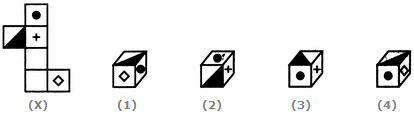Detailed Solution for Cubes And Dice Quiz I, Non Verbal Reasoning - Question 7
The fig. (X) is similar to the Form III. So, when the sheet in fig. (X) is folded to form a cube, then the half-shaded face appears opposite to the face bearing a rhombus, the face with a black circle appears opposite to one of the two blank faces and the face with a '+' sign appears opposite to the other blank face. Clearly, the cubes shown in figures (1) and (4) cannot be formed since they have the half-shaded face adjacent to the face bearing the rhombus. Also, though the cube shown in fig. (3) has faces that can appear adjacent to each other but the cube formed by folding the sheet in fig. (X) cannot be rotated to form fig. (3). Hence, the cube in fig. (3) cannot be formed. Thus, only the cube shown in fig. (2) can be formed.
Cubes And Dice Quiz I, Non Verbal Reasoning - Question 8

The sheet of paper shown in the figure (X) given on the left hand side, in each problem, is folded to form a box. Choose from amongst the alternatives (1), (2), (3) and (4), the boxes that are similar to the box that will be formed.

Question -

Choose the box that is similar to the box formed from the given sheet of paper (X).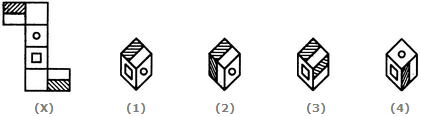Detailed Solution for Cubes And Dice Quiz I, Non Verbal Reasoning - Question 8
The fig. (X) is similar to the Form II. So, when the sheet shown in fig. (X) is folded to form a cube then the two half-shaded faces lie opposite to each other, the face bearing a square lies opposite to one of the two blank faces and the face bearing a circle lies opposite to the other blank face. Therefore, the cubes shown in figures (2) and (3) which have the two half-shaded faces adjacent to each other, cannot be formed by folding the sheet shown in fig. (X). Also, though the cube shown in fig. (4) has faces that can appear adjacent to each other but the cube formed by folding the sheet in fig. (X) cannot be rotated to form the cube in fig. (4). Hence, only the cube in fig. (1) can be formed.
Cubes And Dice Quiz I, Non Verbal Reasoning - Question 9

The sheet of paper shown in the figure (X) given on the left hand side, in each problem, is folded to form a box. Choose from amongst the alternatives (1), (2), (3) and (4), the boxes that are similar to the box that will be formed.

Question -

Choose the box that is similar to the box formed from the given sheet of paper (X).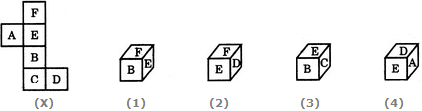Detailed Solution for Cubes And Dice Quiz I, Non Verbal Reasoning - Question 9
The fig. (X) is similar to the Form III. So, when the sheet in fig. (X) is folded to form a cube, then 'F' appears opposite 'B', 'E' appears opposite 'C' and 'A' appears opposite 'D' Therefore, the cube in fig. (1) which shows 'F' adjacent to 'B' the cube in fig. (3) which shows 'E' adjacent to 'C' and the cube in fig. (4) which shows 'A' adjacent to 'D' cannot be formed. Hence, only the cube in fig.(2) can be formed.
Cubes And Dice Quiz I, Non Verbal Reasoning - Question 10

The sheet of paper shown in the figure (X) given on the left hand side, in each problem, is folded to form a box. Choose from amongst the alternatives (1), (2), (3) and (4), the boxes that are similar to the box that will be formed.

Question -

Choose the box that is similar to the box formed from the given sheet of paper (X).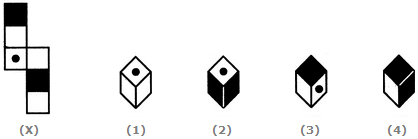Detailed Solution for Cubes And Dice Quiz I, Non Verbal Reasoning - Question 10
The fig. (X) is similar to the Form V. So, when the sheet in fig. (X) is folded to form a cube, then the face bearing a dot lies opposite to one of the shaded faces. Therefore, the cube shown in fig. (2) which has both the shaded faces adjacent to the face bearing the dot, cannot be formed. Hence, the cubes shown in figures (1), (2) and (4) can be formed.
Cubes And Dice Quiz I, Non Verbal Reasoning - Question 11

The sheet of paper shown in the figure (X) given on the left hand side, in each problem, is folded to form a box. Choose from amongst the alternatives (1), (2), (3) and (4), the boxes that are similar to the box that will be formed.

Question -

Choose the box that is similar to the box formed from the given sheet of paper (X).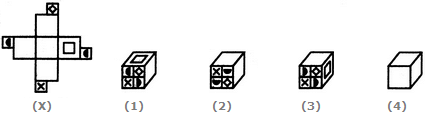Detailed Solution for Cubes And Dice Quiz I, Non Verbal Reasoning - Question 11
The fig. (X) is similar to the Form VII. So, when a cube is formed by folding the sheet shown in fig. (X), then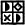is one of the faces of the cube and this face lies opposite to a blank face. Also, a face bearing a square lies opposite to another blank face. The remaining two blank faces lie opposite to each other. Clearly, in the cube shown in fig. (1), the face consisting of the four symbols is not the same as that formed (as shown above). Hence, the cube in fig. (1) cannot be formed.
Cubes And Dice Quiz I, Non Verbal Reasoning - Question 12

The sheet of paper shown in the figure (X) given on the left hand side, in each problem, is folded to form a box. Choose from amongst the alternatives (1), (2), (3) and (4), the boxes that are similar to the box that will be formed.

Question -

Choose the box that is similar to the box formed from the given sheet of paper (X).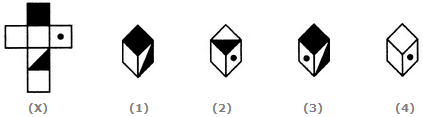Detailed Solution for Cubes And Dice Quiz I, Non Verbal Reasoning - Question 12
The fig. (X) is similar to the Form I. So, when the sheet in fig. (X) is folded to form a cube, then the completely shaded face lies opposite to the half shaded face. Therefore, the cubes shown in figures (1) and (3) which have the completely shaded face adjacent to the half-shaded face cannot be formed. Since Fig 4 does't have at-least one shaded face, it cannot be formed. Hence, only the cubes in figure (2) can be formed.
Cubes And Dice Quiz I, Non Verbal Reasoning - Question 13

The sheet of paper shown in the figure (X) given on the left hand side, in each problem, is folded to form a box. Choose from amongst the alternatives (1), (2), (3) and (4), the boxes that are similar to the box that will be formed.

Question -

Choose the box that is similar to the box formed from the given sheet of paper (X).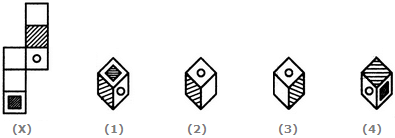Detailed Solution for Cubes And Dice Quiz I, Non Verbal Reasoning - Question 13
The fig. (X) is similar to the Form V. So, when the sheet shown in fig. (X) is folded to form a cube then the shaded face lies opposite to one of the blank faces, the face bearing a circle lies opposite to another blank face and the face bearing a shaded square lies opposite to the third blank face. Thus, each one of the cubes shown in figures (1), (2) and (4) can be formed. Also, though the cube shown in fig. (3) has faces that can appear adjacent to each other but the cube formed by folding the sheet in fig. (X) cannot be rotated to form fig. (3). Hence, the cube in fig.(3) cannot be formed.
Cubes And Dice Quiz I, Non Verbal Reasoning - Question 14

The sheet of paper shown in the figure (X) given on the left hand side, in each problem, is folded to form a box. Choose from amongst the alternatives (1), (2), (3) and (4), the boxes that are similar to the box that will be formed.

Question -

Choose the box that is similar to the box formed from the given sheet of paper (X).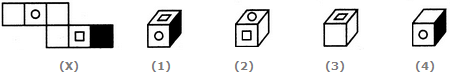Detailed Solution for Cubes And Dice Quiz I, Non Verbal Reasoning - Question 14
The fig. (X) is similar to the Form V. So, when the sheet in fig. (X) is folded to form a cube, then the face bearing a square lies opposite to the face bearing a circle. Therefore, the cubes shown in figures (1) and (2) which have the faces bearing the square and the circle adjacent to each other, cannot be formed. Hence, only the cubes in figures (3) and (4) can be formed.
Cubes And Dice Quiz I, Non Verbal Reasoning - Question 15

The sheet of paper shown in the figure (X) given on the left hand side, in each problem, is folded to form a box. Choose from amongst the alternatives (1), (2), (3) and (4), the boxes that are similar to the box that will be formed.

Question -

Choose the box that is similar to the box formed from the given sheet of paper (X).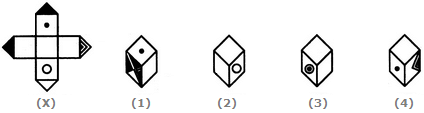Detailed Solution for Cubes And Dice Quiz I, Non Verbal Reasoning - Question 15
The fig. (X) is similar to the Form VI. So, when a cube is formed by folding the sheet shown in fig. (X), then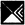is one of the faces of the cube and this face lies opposite to a blank face. Also, a face bearing a circle lies opposite to one bearing a dot. Clearly, this cube does not have faces as shown in the cubes in figures (3) and (4). Hence, only the cubes shown in figures (1) and (2) can be formed.
Cubes And Dice Quiz I, Non Verbal Reasoning - Question 16

The sheet of paper shown in the figure (X) given on the left hand side, in each problem, is folded to form a box. Choose from amongst the alternatives (1), (2), (3) and (4), the boxes that are similar to the box that will be formed.

Question -

Choose the box that is similar to the box formed from the given sheet of paper (X).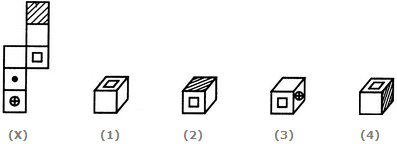Detailed Solution for Cubes And Dice Quiz I, Non Verbal Reasoning - Question 16
The fig. (X) is similar to Form V. So, when the sheet shown in fig. (X) is folded to form a cube, then the face with shading lies opposite to the free bearing a square, the face bearing a dot lies opposite to a blank face and the face bearing a circle (with a '+' sign inside it) lies opposite to another blank face. The cubes in figures (2) and (4) have the shaded face adjacent to the face bearing a square. Therefore, the cubes in these two figures cannot be formed. Hence, only cubes in figures (1) and (3) can be formed.
Cubes And Dice Quiz I, Non Verbal Reasoning - Question 17

The sheet of paper shown in the figure (X) given on the left hand side, in each problem, is folded to form a box. Choose from amongst the alternatives (1), (2), (3) and (4), the boxes that are similar to the box that will be formed.

Question -

Which of the following finished patterns can be obtained from the piece of cardboard (X) shown below?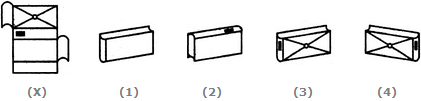Detailed Solution for Cubes And Dice Quiz I, Non Verbal Reasoning - Question 17
The pattern on fig. (X) and also the fact that the faces are rectangle, indicate that only fig. (1) can be obtained by folding fig. (X).
Cubes And Dice Quiz I, Non Verbal Reasoning - Question 18

The sheet of paper shown in the figure (X) given on the left hand side, in each problem, is folded to form a box. Choose from amongst the alternatives (1), (2), (3) and (4), the boxes that are similar to the box that will be formed.

Question -

Choose the box that is similar to the box formed from the given sheet of paper (X).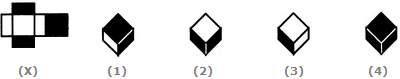Detailed Solution for Cubes And Dice Quiz I, Non Verbal Reasoning - Question 18
The fig. (X) is similar to Form I. So, when the sheet shown in fig. (X) is folded to form a box (cuboid), then the two rectangular-shaded faces lie opposite to each other, two rectangular white faces lie opposite to each other and the two square shaped faces (one shaded and one white) lie opposite to each other. Clearly, the cuboids shown in figures (2) and (4) cannot be formed as in each of the two cuboids the two shaded rectangular faces appear adjacent to each other. So, only the cuboids in figures (1) and (3) can be formed.
Cubes And Dice Quiz I, Non Verbal Reasoning - Question 19

The sheet of paper shown in the figure (X) given on the left hand side, in each problem, is folded to form a box. Choose from amongst the alternatives (1), (2), (3) and (4), the boxes that are similar to the box that will be formed.

Question -

Choose the box that is similar to the box formed from the given sheet of paper (X).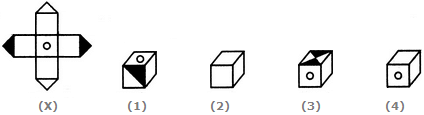Detailed Solution for Cubes And Dice Quiz I, Non Verbal Reasoning - Question 19

The fig. (X) is similar to the Form VI. So, when the cube is formed by folding the sheet shown in fig. (X), then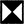is one of the faces of the cube and this face lies opposite to the face bearing a circle. Also, one of the blank faces lies opposite to another blank face and yet another blank face lies opposite to the fourth blank face. Thus, out of the four blank faces, no three faces can appear adjacent to each other.

Clearly, the cube in fig. (1) cannot be formed since there is no face of the type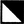, the cube in fig. (2) cannot be formed since it shows three blank faces adjacent to each other and the cube in fig. (3) cannot be formed since the face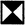cannot appear adjacent to the face bearing the circle. Hence, only the cube in fig. (4) can be formed.

Cubes And Dice Quiz I, Non Verbal Reasoning - Question 20

The sheet of paper shown in the figure (X) given on the left hand side, in each problem, is folded to form a box. Choose from amongst the alternatives (1), (2), (3) and (4), the boxes that are similar to the box that will be formed.

Question -

When the following figure is folded to form a cube, how many dots lie opposite the face bearing five dots?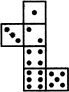Detailed Solution for Cubes And Dice Quiz I, Non Verbal Reasoning - Question 20
The given figure is similar to Form III. Therefore, when this figure is folded to form a cube then the face bearing three dots will lie opposite the face bearing five dots.
Cubes And Dice Quiz I, Non Verbal Reasoning - Question 21

The sheet of paper shown in the figure (X) given on the left hand side, in each problem, is folded to form a box. Choose from amongst the alternatives (1), (2), (3) and (4), the boxes that are similar to the box that will be formed.

Question -

Choose the box that is similar to the box formed from the given sheet of paper (X).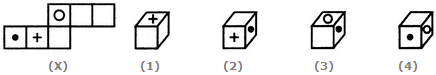Detailed Solution for Cubes And Dice Quiz I, Non Verbal Reasoning - Question 21
The fig. (X) is similar to the Form V. So, when the sheet in fig. (X) is folded to form a cube, then the face bearing a dot appears opposite to a blank face, the face bearing a '+' sign appears opposite to another blank face and the face bearing a circle appears opposite to the third blank face. Clearly, all the four cubes shown in figures (1), (2), (3) and (4) can be formed.
Cubes And Dice Quiz I, Non Verbal Reasoning - Question 22

The sheet of paper shown in the figure (X) given on the left hand side, in each problem, is folded to form a box. Choose from amongst the alternatives (1), (2), (3) and (4), the boxes that are similar to the box that will be formed.

Question -

Choose the box that is similar to the box formed from the given sheet of paper (X).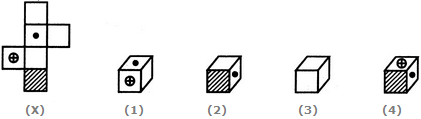Detailed Solution for Cubes And Dice Quiz I, Non Verbal Reasoning - Question 22
The fig. (X) is similar to Form IV. So, when the sheet shown in fig. (X) is folded to , form a cube, then the face bearing the dot lies opposite to the shaded face, the face bearing a circle (with '+' sign inside it) lies opposite to a blank face and the remaining two blank faces lie opposite to each other. Clearly, the cubes shown in figures (2) and (4) cannot be formed since they have the shaded face adjacent to the face bearing a dot and the cube shown in fig. (3) cannot be formed since it shows all the three blank faces adjacent to each other. Hence only the cube shown in fig. (1) can be formed.
Cubes And Dice Quiz I, Non Verbal Reasoning - Question 23

The sheet of paper shown in the figure (X) given on the left hand side, in each problem, is folded to form a box. Choose from amongst the alternatives (1), (2), (3) and (4), the boxes that are similar to the box that will be formed.

Question -

Choose the box that is similar to the box formed from the given sheet of paper (X).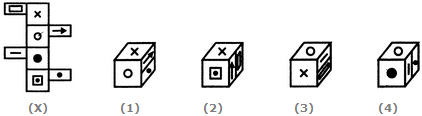Detailed Solution for Cubes And Dice Quiz I, Non Verbal Reasoning - Question 23
The fig. (X) is similar to the Form VIII. So, when a cube is formed by folding the sheet shown in fig. (X), then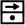and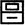are the two faces and these two faces lie opposite to each other. Also, the face bearing the 'x' sigh lies opposite to the face bearing the black circle and the face bearing the white circle lies opposite to the face bearing the square (having a dot inside it). Now, the cubes in figures (2) and (4) consist of faces which are not formed when the sheet in fig. (X) is folded. Hence, these two cubes are not, formed. Therefore, only the cubes in figures (1) and (3) are formed.
Cubes And Dice Quiz I, Non Verbal Reasoning - Question 24

Observe the dots on a dice (one to six dots) in the following figures. How many dots are contained on the face opposite to that containing four dots?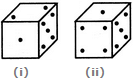Detailed Solution for Cubes And Dice Quiz I, Non Verbal Reasoning - Question 24

We shall assume the dice in fig. (ii) to be rotated so that the 5 dots appear at the same position as in fig. (i) i.e. on RHS face (i.e. on face II as per activity 1) and 1 dot appears at the same position as in fig; (i) i.e. on Front face (i.e. on face I). Then, from the, two figures, 2 dots appear on the top face (i.e. on face V) and 4 dots appear on the Bottom face (i.e. on face VI).

Since, these two faces are opposite to each other, therefore, two dots are contained on the face opposite to that containing four dots.

Cubes And Dice Quiz I, Non Verbal Reasoning - Question 25

Three different positions of a dice are shown below. How many dots lie opposite 2 dots?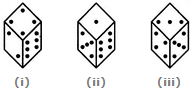Detailed Solution for Cubes And Dice Quiz I, Non Verbal Reasoning - Question 25

From figures (ii) and (iii), we conclude that 1, 6, 3 and 4 dots lie adjacent to 5 dots. Therefore, 2 dots must lie opposite 5 dots. Conversely, 5 dots must lie opposite 2 dots.

## Non Verbal Reasoning

40 tests
Information about Cubes And Dice Quiz I, Non Verbal Reasoning Page
In this test you can find the Exam questions for Cubes And Dice Quiz I, Non Verbal Reasoning solved & explained in the simplest way possible. Besides giving Questions and answers for Cubes And Dice Quiz I, Non Verbal Reasoning, EduRev gives you an ample number of Online tests for practice

40 tests